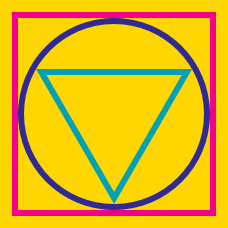Geometry

# Circumcircle of Squares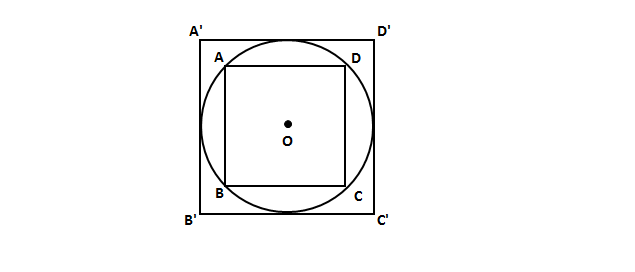In the above diagram, square $ABCD$ is inscribed in circle $O,$ which is in turn inscribed in square $A'B'C'D'.$ If the length of $\overline{A'D'}$ is $\lvert\overline{A'D'}\rvert=8,$ what is $\lvert\overline{AD}\rvert?$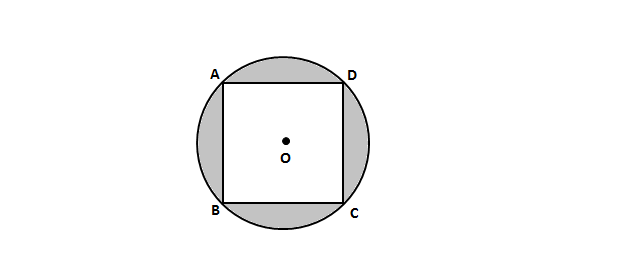The area of the square inscribed in circle $O$ in the above diagram is $484.$ What is the area of the shaded region?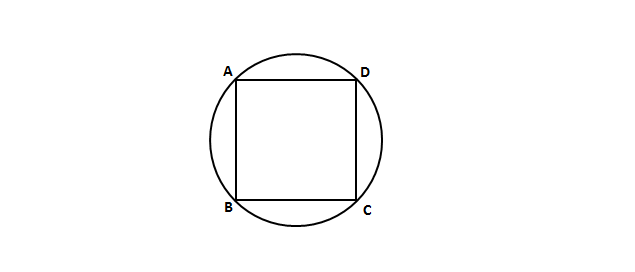The area of the circle in the above diagram is $64\pi.$ What is the area of the inscribed square $ABCD?$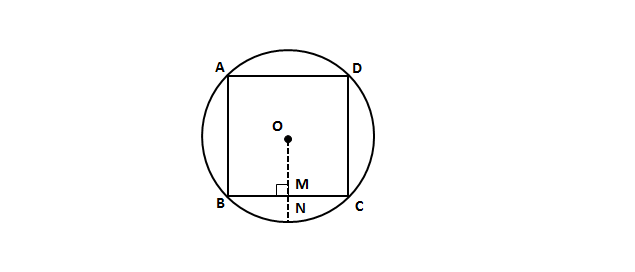In the above diagram, $\square ABCD$ is a square inscribed in circle $O,$ and $\overline{ON}$ is perpendicular to $\overline{BC}.$ If the length of $\overline{MN}$ is $2\sqrt{2}-2,$ what is the length of $\overline{BM}?$

For which of the following quadrilaterals does a circumscribing circle always exist?

×